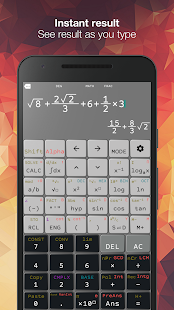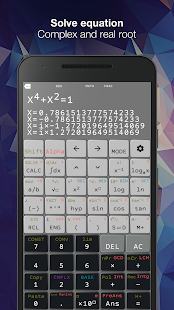# N-CALC – FX 570 ES/VN PLUS v2.0.9 [Premium] ApkN-CALC
Natural Scientific Calculator for Android

Natural display
Other apps make you enter equations on a single line like something from the 1970s. Our Natural Input solves this problem by allowing you to enter equations as you’d write them on paper, using fractions, roots, exponents and more. It’s called a “natural display” and is a common feature on scientific calculators like the Casio fx 570 991 es/vn plus, and we’ve finally brought it over to Android!

Confused when your equation looks like this? 50∗1/(0.05/12−0.08/12)[1−((1+0.08/12)/(1+0.05/12))^3]. We are too, so we’ve made structures like fractions display naturally as it does on paper.

Scroll featureSwipe on the keyboard to move the cursor up and down, left and right. Touch to edit expression.

Calculate functionality• Solve equation
• Derivative function
• Integrate function
• Base N calculation
• Table
• Solve cubic polynomial
• Complex number

Output type
• Fraction
• Mixed fraction
• Decimal
• Repeat decimal
• Degree/Minus/Second
• Polar coordinate

More feature• Automatic calculate: see result as you typing
• Vibrate and sound
• Decimal formatter
• Calculation history
• Variable value
• Full keyboard and compact keyboard
• Change font family
• Change theme
• Change font size

What’s New
Svenska language

Screenshots

••